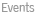backSeptember, 18 - Boris M. Schein (University of Arkansas)
 Ifis a semigroup of functions (= partial transformations) in a setthenis (partially) ordered in a natural way. Ifthenprecisely when, that is, for every, ifis defined thenis defined and. Every (abstract) semigroupis isomorphic to various semigroupsof functions on various sets. Under this isomorphism,is ordered by an order relation isomorphic torelation on. These order relations onare called fundamental. Thus, fundamental orders ofare an important subclass of all possible order relations on. They reflect certain specific properties ofwhen this semigroup is viewed as a semigroup of functions. Very much depends on what we mean by functions". The most general class of functions is binary relations between the elements of. Ifis such a relation (that is,) andfor some, thenis an image" ofwith respect to. Thusis a many-valued function, eachmay have many (or no) images. Or we may consider ordinary functions (eachhas at most one image under). Or we may consider one-to-one partial transformations only, etc. The wider is the class of our functions", the more fundamental orderings there may exist on our semigroup. The speaker will characterize fundamental order relations on abstract semigroups giving simple necessary and sufficient conditions for each order relaton to be fundamental. back© 2021 Universidade do Minho  - Legal Terms  - updated by CMATD.